Example Questions

Example Question #51 : Arithmetic

Solve: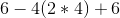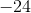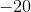Explanation:

In PEMDAS, we apply parentheses first, followed by multiplication and finally subtraction and addition from left to right.

Therefore,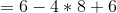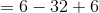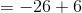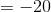Example Question #52 : Arithmetic

Solve: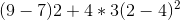Explanation:

In PEMDAS, parentheses from left to right, followed by exponents, multiplication from left to right, and finally addition.

Therefore,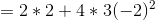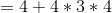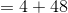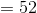Example Question #53 : Arithmetic

Solve: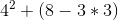Explanation:

In PEMDAS, parentheses is applied with multiplication first followed by subtraction and finally do the exponents and addition.

Therefore,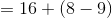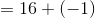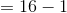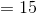Example Question #54 : Arithmetic

Solve: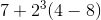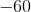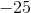Explanation:

In PEMDAS, the exponent is applied first, followed by parentheses, then multiplication and lastly addition.

Therefore,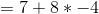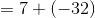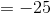Example Question #55 : Arithmetic

If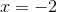and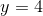, then what is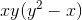?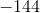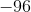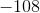Explanation:Apply substitution.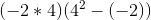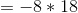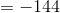Example Question #56 : Arithmetic

Ifand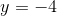, then what is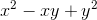?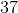Explanation:Apply substitution.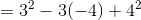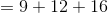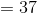Example Question #57 : Arithmetic

Consider the expression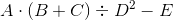If the values of all of the variables are known, which operation is carried out third?

Squaring

Multiplication

Division

Subtraction

Multiplication

Explanation:

The order of operations dictates that any operations within grouping symbols, such as parentheses, be carried out first; in this expression, this is the addition.

This leaves a multiplication, a division, an exponentiation (squaring), and a subtraction. The squaring takes precedence over the others, so it is carried out second. Multiplication and division take precedence over subtraction; since the multiplication is first in left-to-right order, it is carried out third. This makes multiplication the correct response.

Example Question #58 : Arithmetic

Consider the expression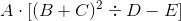If the values of all of the variables are known, which operation is carried out third?

Division

Squaring

Multiplication

Subtraction

Division

Explanation:

The order of operations dictates that any operations within grouping symbols is carried out first, going from the innermost symbols to the outermost symbols. The innermost symbols - the parentheses - flank an addition, so that operation is carried out first.

The operations between the outermost symbols - the brackets - are carried out next. There is an exponentiation (squaring), a division, and a subtraction there; by order of operations, the squaring is carried out next, and the division after that. Division is therefore the correct response.

Example Question #59 : Arithmetic

Consider the expression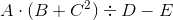If the values of all of the variables are known, which operation is carried out third?

Division

Multiplication

Squaring

Subtraction

Multiplication

Explanation:

The order of operations dictates that any operations within grouping symbols, such as parentheses, be carried out first; in this expression. There are two expressions - an exponentiation (squaring) and an addition - that fall between the parentheses; by the order of operations, the squaring is carried out, then the addition.

This leaves a multiplication, a division, and a subtraction. Multiplication and division have precedence over subtraction; since the multiplication appears first in left-to-right order, the multiplication is carried out next. This makes multiplication the correct response.

Example Question #60 : Arithmetic

Consider the expression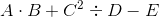If the values of all of the variables are known, which operation is carried out third?

Multiplication

Division

Squaring

Subtraction